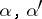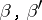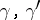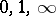Riemann differential equation

A linear homogeneous ordinary differential equation of the second order in the complex plane with three given regular singular points (cf. Regular singular point),andhaving characteristic exponents,,at these points. The general form of such an equation was first given by E. Papperitz, because of which it is also known as a Papperitz equation. Solutions of a Riemann differential equation are written in the form of the so-called Riemann-functionRiemann differential equations belong to the class of Fuchsian equations (cf. Fuchsian equation) with three singular points. A particular case of Riemann differential equations is the hypergeometric equation (the singular points are); therefore, a Riemann differential equation itself is sometimes known as a generalized hypergeometric equation. A Riemann differential equation can be reduced to a Pochhammer equation, and its solution can thus be written in the form of an integral over a special contour in the complex plane.